# The graphs of $y = p(x)$ are given in figure below, for some polynomials $p(x)$. Find the number of zeroes of $p(x)$, in each case.(i)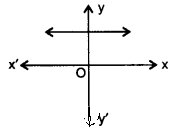(ii)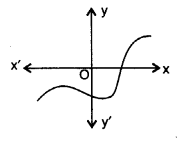(iii)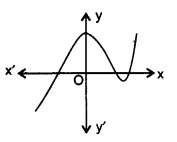(iv)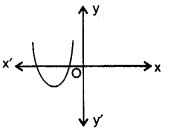(v)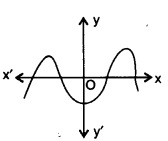(vi)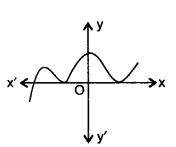"

To do:

We have to find the number of zeroes of $p(x)$ in each case.

Solution:

(i) The given graph does not touch the x-axis at any point. Thus, the number of zeroes is $0$.

(ii) The graph touches the x-axis at one point. Thus, the number of zeroes is $1$.

(iii) The graph touches the x-axis at three points. Thus, the number of zeroes is $3$.

(iv) The graph touches the x-axis at two points. Thus, the number of zeroes is $2$.

(v) The graph touches the x-axis at $4$ points. Thus, the number of zeroes is $4$.

(vi) The graph touches the x-axis at $3$ points. Thus, the number of zeroes is $3$.